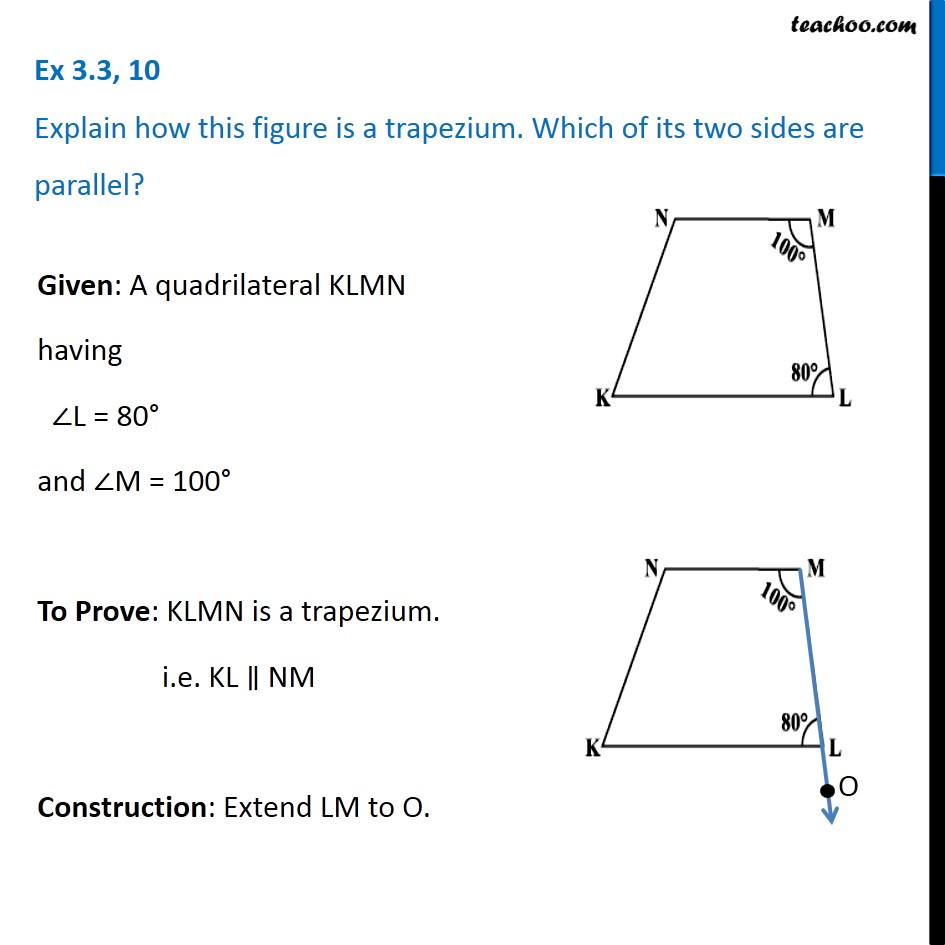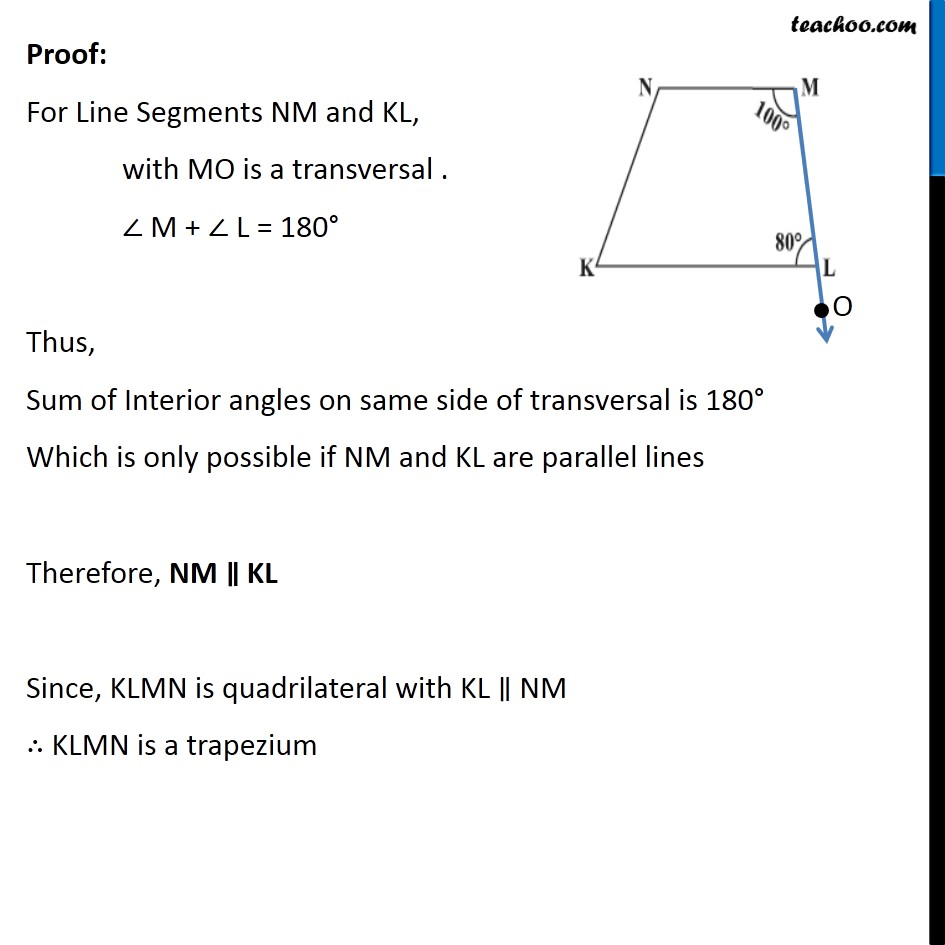Chapter 3 Class 8 Understanding Quadrilaterals
Concept wiseLearn in your speed, with individual attention - Teachoo Maths 1-on-1 Class

### Transcript

Ex 3.3, 10 Explain how this figure is a trapezium. Which of its two sides are parallel? Given: A quadrilateral KLMN having ∠L = 80° and ∠M = 100° To Prove: KLMN is a trapezium. i.e. KL ∥ NM Construction: Extend LM to O. Proof: For Line Segments NM and KL, with MO is a transversal . ∠ M + ∠ L = 180° Thus, Sum of Interior angles on same side of transversal is 180° Which is only possible if NM and KL are parallel lines Therefore, NM ∥ KL Since, KLMN is quadrilateral with KL ∥ NM ∴ KLMN is a trapezium